### [SQL Server] Querying Data in a Graph Database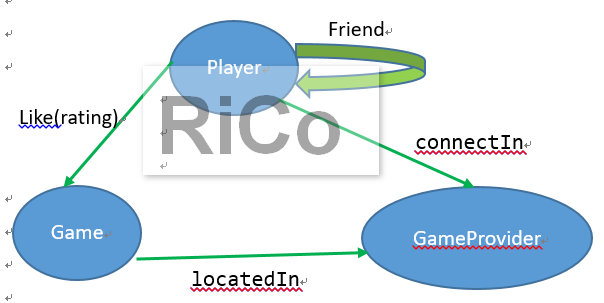--玩家
create table player (
id int primary key,
name nvarchar(100)
) as node;

--遊戲清單
drop table game
create table game (
id int not null,
name varchar(100),
gameprovidername varchar(100)
) as node;

--遊戲供應商
create table gameprovider (
id int primary key,
name varchar(100),
country varchar(100)
) as node;

--建立edge 資料表
CREATE TABLE likes (rating int) AS EDGE;
CREATE TABLE friendOf AS EDGE;
CREATE TABLE connectIn AS EDGE;
CREATE TABLE locatedIn AS EDGE;

-- Insert data into node tables.
INSERT INTO player VALUES (1,'ricoisme');
INSERT INTO player VALUES (2,'rico');
INSERT INTO player VALUES (3,'sherry');
INSERT INTO player VALUES (4,'fifi');
INSERT INTO player VALUES (5,'pcdou');

INSERT INTO game VALUES (1,'Love Fish','RT');
INSERT INTO game VALUES (2,'MJ 13','BG');
INSERT INTO game VALUES (3,'Point 21', 'GY');

INSERT INTO gameprovider VALUES (1,'RT','eg');
INSERT INTO gameprovider VALUES (2,'BG','eg');
INSERT INTO gameprovider VALUES (3,'GY','it');

-- Insert into edge table. While inserting into an edge table,
-- you need to provide the $node_id from$from_id and $to_id columns. INSERT INTO likes VALUES ((SELECT$node_id FROM player WHERE id = 1),
(SELECT $node_id FROM game WHERE id = 1),9); INSERT INTO likes VALUES ((SELECT$node_id FROM player WHERE id = 2),
(SELECT $node_id FROM game WHERE id = 2),9); INSERT INTO likes VALUES ((SELECT$node_id FROM player WHERE id = 3),
(SELECT $node_id FROM game WHERE id = 3),9); INSERT INTO likes VALUES ((SELECT$node_id FROM player WHERE id = 4),
(SELECT $node_id FROM game WHERE id = 3),9); INSERT INTO likes VALUES ((SELECT$node_id FROM player WHERE id = 4),
(SELECT $node_id FROM game WHERE id = 2),9); INSERT INTO likes VALUES ((SELECT$node_id FROM player WHERE id = 5),
(SELECT $node_id FROM game WHERE id = 3),9); INSERT INTO likes VALUES ((SELECT$node_id FROM player WHERE id = 2),
(SELECT $node_id FROM game WHERE id = 3),9); INSERT INTO likes VALUES ((SELECT$node_id FROM player WHERE id = 2),
(SELECT $node_id FROM game WHERE id = 1),9); INSERT INTO connectIn VALUES ((SELECT$node_id FROM player WHERE id = 1),
(SELECT $node_id FROM gameprovider WHERE id = 1)); INSERT INTO connectIn VALUES ((SELECT$node_id FROM player WHERE id = 2),
(SELECT $node_id FROM gameprovider WHERE id = 2)); INSERT INTO connectIn VALUES ((SELECT$node_id FROM player WHERE id = 3),
(SELECT $node_id FROM gameprovider WHERE id = 3)); INSERT INTO connectIn VALUES ((SELECT$node_id FROM player WHERE id = 4),
(SELECT $node_id FROM gameprovider WHERE id = 3)); INSERT INTO connectIn VALUES ((SELECT$node_id FROM player WHERE id = 5),
(SELECT $node_id FROM gameprovider WHERE id = 1)); INSERT INTO locatedIn VALUES ((SELECT$node_id FROM game WHERE id = 1),
(SELECT $node_id FROM gameprovider WHERE id =1)); INSERT INTO locatedIn VALUES ((SELECT$node_id FROM game WHERE id = 2),
(SELECT $node_id FROM gameprovider WHERE id =2)); INSERT INTO locatedIn VALUES ((SELECT$node_id FROM game WHERE id = 3),
(SELECT $node_id FROM gameprovider WHERE id =3)); -- Insert data into the friendof edge. INSERT INTO friendOf VALUES ((SELECT$NODE_ID FROM player WHERE ID = 1), (SELECT $NODE_ID FROM player WHERE ID = 2)); INSERT INTO friendOf VALUES ((SELECT$NODE_ID FROM player WHERE ID = 2), (SELECT $NODE_ID FROM player WHERE ID = 3)); INSERT INTO friendOf VALUES ((SELECT$NODE_ID FROM player WHERE ID = 3), (SELECT $NODE_ID FROM player WHERE ID = 1)); INSERT INTO friendOf VALUES ((SELECT$NODE_ID FROM player WHERE ID = 4), (SELECT $NODE_ID FROM player WHERE ID = 2)); INSERT INTO friendOf VALUES ((SELECT$NODE_ID FROM player WHERE ID = 5), (SELECT \$NODE_ID FROM player WHERE ID = 4));

-- 查詢 rico 有玩過的遊戲
SELECT distinct game.name
FROM player, likes, game
WHERE MATCH (player-(likes)->game)
AND player.name = 'rico';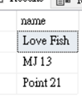-- 查詢 rico 的好友
SELECT player1.name,[Good Friend]=player2.name
FROM player player1, friendOf, player player2
WHERE MATCH (player1-(friendOf)->player2)
AND player1.name='rico';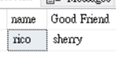-- 查詢 rico 的朋友最喜愛遊戲
SELECT player2.name, game.name
FROM player player1, player player2, likes, friendOf, game
WHERE MATCH(player1-(friendOf)->player2-(likes)->game)
AND player1.name='rico';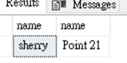--查詢連到相同地區的所有玩家
SELECT player.name,game.name,gameprovider.country
FROM player, likes, game, gameprovider, locatedIn,connectIn
WHERE MATCH (player-(likes)->game-(locatedIn)->gameprovider AND player-(connectIn)->gameprovider );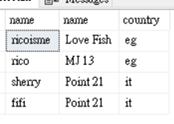Let's start SQL Server Graph Database